David Starshaw

•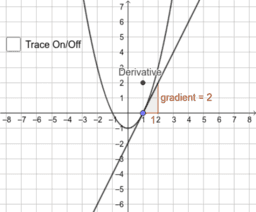Differentiation is Gradient at a Point

Activity

David Starshaw

•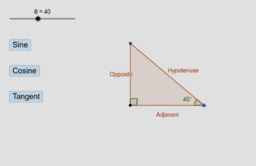What Sine, Cosine, and Tangent Actually Mean

Activity

David Starshaw

•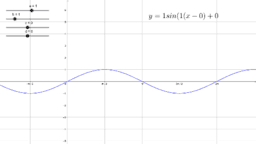Transformations of trigonometric graphs

Activity

David Starshaw

•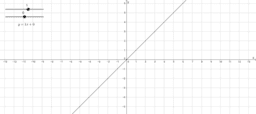Drawing graphs from linear equations

Activity

David Starshaw

•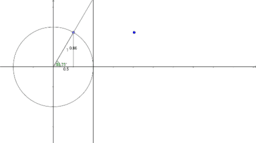Sine trig ratio

Activity

David Starshaw

•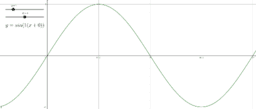Horizontal transformations

Activity

David Starshaw

•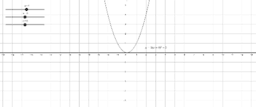Parabolas (completed square form)

Activity

David Starshaw

•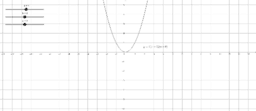Parabolas (factorised form)

Activity

David Starshaw

•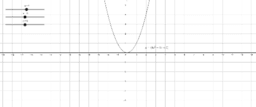Parabolas (expanded form)

Activity

David Starshaw

•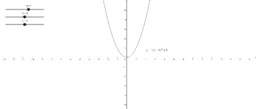Parabolas (completed square form)

Activity

David Starshaw

•Parabolas (completed square form)

Activity

David Starshaw

•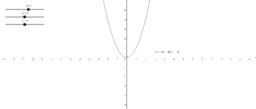Parabolas (factorised form)

Activity

David Starshaw

•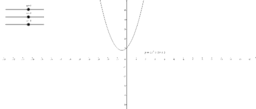Parabolas (expanded form)

Activity

David Starshaw

•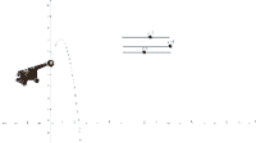Activity

David Starshaw

•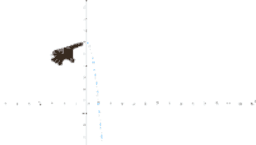Activity

David Starshaw

•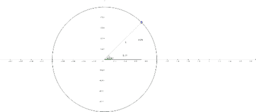Unit circle

Activity

David Starshaw

•Unit circle

Activity

David Starshaw